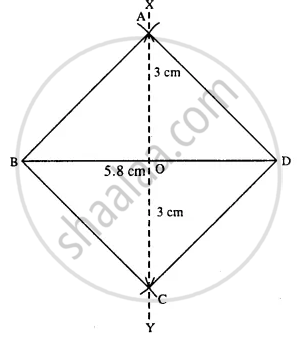# Construct a Rhombus Abcd, If ; Diagonal Ac = 6 Cm and Diagonal Bd = 5.8 Cm. - Mathematics

Sum

Construct a rhombus ABCD, if ;

diagonal AC = 6 cm and diagonal BD = 5.8 cm.

#### SolutionSteps :

1. Draw BD = 5.8 cm.
2. Draw perpendicular bisector XY of BD.
3. Cut OA = OC = 3 cm (half the diagonal 6 cm)
4. Join AB, AD, BC, and CD.
Thus ABCD is the required rhombus.
Concept: Construction of Rhombus
Is there an error in this question or solution?

#### APPEARS IN

Selina Concise Mathematics Class 8 ICSE
Chapter 18 Constructions
Exercise 18 (D) | Q 4.5 | Page 211